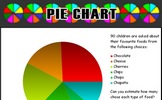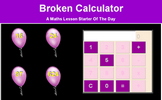# Statistics

### Term 3 starting in week 1 :: Estimated time: 2 weeks

• Read and interpret line graphs
• Draw line graphs
• Use line graphs to solve problems
• Circles
• Read and interpret pie charts
• Pie charts with percentages
• Draw pie charts
• The mean

This page should remember your ticks from one visit to the next for a period of time. It does this by using Local Storage so the information is saved only on the computer you are working on right now.

## Lesson Starters

Here are some suggestions for whole-class, projectable resources which can be used at the beginnings of each lesson in this block.

### 1st Lesson#### Average Smile

Calculate the mean, median and range of the given scores.

### 2nd Lesson#### Pie Chart

An exercise in estimating what the sectors of a pie chart represent.

### 3rd LessonAdd up a sequence of consecutive numbers. Can you find a quick way to do it?

### 4th Lesson#### Balloon Bursting Buttons

Use only the 1, 5 and 0 keys on a calculator to make given totals.

### 5th Lesson#### Four Factors

Find four single digit numbers that multiply together to give 120. How many different ways are there of answering this question?

### 6th LessonStand at the point between the classroom walls to represent a given number.

Some of the Starters above are to reinforce concepts learnt, others are to introduce new ideas while others are on unrelated topics designed for retrieval practice or and opportunity to develop problem-solving skills.

White Rose ResourcesEnd of block assessments provide a quick progress check at the end of each block of learning to make sure students have understood the content covered. This Scheme of Learning was produced by White Rose Maths and is used here with permission granted on 30th June 2021.For All: